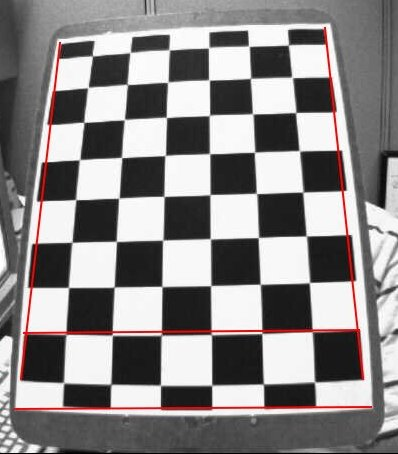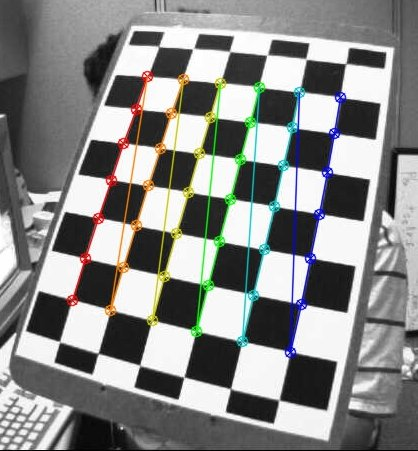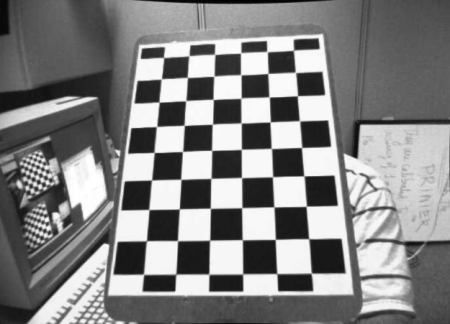OpenCV  3.4.18-dev Open Source Computer Vision
Camera Calibration

## Goal

In this section, we will learn about

• types of distortion caused by cameras
• how to find the intrinsic and extrinsic properties of a camera
• how to undistort images based off these properties

## Basics

Some pinhole cameras introduce significant distortion to images. Two major kinds of distortion are radial distortion and tangential distortion.

Radial distortion causes straight lines to appear curved. Radial distortion becomes larger the farther points are from the center of the image. For example, one image is shown below in which two edges of a chess board are marked with red lines. But, you can see that the border of the chess board is not a straight line and doesn't match with the red line. All the expected straight lines are bulged out. Visit Distortion (optics) for more details.image

Radial distortion can be represented as follows:

$x_{distorted} = x( 1 + k_1 r^2 + k_2 r^4 + k_3 r^6) \\ y_{distorted} = y( 1 + k_1 r^2 + k_2 r^4 + k_3 r^6)$

Similarly, tangential distortion occurs because the image-taking lense is not aligned perfectly parallel to the imaging plane. So, some areas in the image may look nearer than expected. The amount of tangential distortion can be represented as below:

$x_{distorted} = x + [ 2p_1xy + p_2(r^2+2x^2)] \\ y_{distorted} = y + [ p_1(r^2+ 2y^2)+ 2p_2xy]$

In short, we need to find five parameters, known as distortion coefficients given by:

$Distortion \; coefficients=(k_1 \hspace{10pt} k_2 \hspace{10pt} p_1 \hspace{10pt} p_2 \hspace{10pt} k_3)$

In addition to this, we need to some other information, like the intrinsic and extrinsic parameters of the camera. Intrinsic parameters are specific to a camera. They include information like focal length ( $$f_x,f_y$$) and optical centers ( $$c_x, c_y$$). The focal length and optical centers can be used to create a camera matrix, which can be used to remove distortion due to the lenses of a specific camera. The camera matrix is unique to a specific camera, so once calculated, it can be reused on other images taken by the same camera. It is expressed as a 3x3 matrix:

$camera \; matrix = \left [ \begin{matrix} f_x & 0 & c_x \\ 0 & f_y & c_y \\ 0 & 0 & 1 \end{matrix} \right ]$

Extrinsic parameters corresponds to rotation and translation vectors which translates a coordinates of a 3D point to a coordinate system.

For stereo applications, these distortions need to be corrected first. To find these parameters, we must provide some sample images of a well defined pattern (e.g. a chess board). We find some specific points of which we already know the relative positions (e.g. square corners in the chess board). We know the coordinates of these points in real world space and we know the coordinates in the image, so we can solve for the distortion coefficients. For better results, we need at least 10 test patterns.

## Code

As mentioned above, we need at least 10 test patterns for camera calibration. OpenCV comes with some images of a chess board (see samples/data/left01.jpg – left14.jpg), so we will utilize these. Consider an image of a chess board. The important input data needed for calibration of the camera is the set of 3D real world points and the corresponding 2D coordinates of these points in the image. 2D image points are OK which we can easily find from the image. (These image points are locations where two black squares touch each other in chess boards)

What about the 3D points from real world space? Those images are taken from a static camera and chess boards are placed at different locations and orientations. So we need to know $$(X,Y,Z)$$ values. But for simplicity, we can say chess board was kept stationary at XY plane, (so Z=0 always) and camera was moved accordingly. This consideration helps us to find only X,Y values. Now for X,Y values, we can simply pass the points as (0,0), (1,0), (2,0), ... which denotes the location of points. In this case, the results we get will be in the scale of size of chess board square. But if we know the square size, (say 30 mm), we can pass the values as (0,0), (30,0), (60,0), ... . Thus, we get the results in mm. (In this case, we don't know square size since we didn't take those images, so we pass in terms of square size).

3D points are called object points and 2D image points are called image points.

### Setup

So to find pattern in chess board, we can use the function, cv.findChessboardCorners(). We also need to pass what kind of pattern we are looking for, like 8x8 grid, 5x5 grid etc. In this example, we use 7x6 grid. (Normally a chess board has 8x8 squares and 7x7 internal corners). It returns the corner points and retval which will be True if pattern is obtained. These corners will be placed in an order (from left-to-right, top-to-bottom)

Note
This function may not be able to find the required pattern in all the images. So, one good option is to write the code such that, it starts the camera and check each frame for required pattern. Once the pattern is obtained, find the corners and store it in a list. Also, provide some interval before reading next frame so that we can adjust our chess board in different direction. Continue this process until the required number of good patterns are obtained. Even in the example provided here, we are not sure how many images out of the 14 given are good. Thus, we must read all the images and take only the good ones.
Instead of chess board, we can alternatively use a circular grid. In this case, we must use the function cv.findCirclesGrid() to find the pattern. Fewer images are sufficient to perform camera calibration using a circular grid.

Once we find the corners, we can increase their accuracy using cv.cornerSubPix(). We can also draw the pattern using cv.drawChessboardCorners(). All these steps are included in below code:

import numpy as np
import cv2 as cv
import glob
# termination criteria
criteria = (cv.TERM_CRITERIA_EPS + cv.TERM_CRITERIA_MAX_ITER, 30, 0.001)
# prepare object points, like (0,0,0), (1,0,0), (2,0,0) ....,(6,5,0)
objp = np.zeros((6*7,3), np.float32)
objp[:,:2] = np.mgrid[0:7,0:6].T.reshape(-1,2)
# Arrays to store object points and image points from all the images.
objpoints = [] # 3d point in real world space
imgpoints = [] # 2d points in image plane.
images = glob.glob('*.jpg')
for fname in images:
gray = cv.cvtColor(img, cv.COLOR_BGR2GRAY)
# Find the chess board corners
ret, corners = cv.findChessboardCorners(gray, (7,6), None)
# If found, add object points, image points (after refining them)
if ret == True:
objpoints.append(objp)
corners2 = cv.cornerSubPix(gray,corners, (11,11), (-1,-1), criteria)
imgpoints.append(corners2)
# Draw and display the corners
cv.drawChessboardCorners(img, (7,6), corners2, ret)
cv.imshow('img', img)

One image with pattern drawn on it is shown below:image

### Calibration

Now that we have our object points and image points, we are ready to go for calibration. We can use the function, cv.calibrateCamera() which returns the camera matrix, distortion coefficients, rotation and translation vectors etc.

ret, mtx, dist, rvecs, tvecs = cv.calibrateCamera(objpoints, imgpoints, gray.shape[::-1], None, None)

### Undistortion

Now, we can take an image and undistort it. OpenCV comes with two methods for doing this. However first, we can refine the camera matrix based on a free scaling parameter using cv.getOptimalNewCameraMatrix(). If the scaling parameter alpha=0, it returns undistorted image with minimum unwanted pixels. So it may even remove some pixels at image corners. If alpha=1, all pixels are retained with some extra black images. This function also returns an image ROI which can be used to crop the result.

So, we take a new image (left12.jpg in this case. That is the first image in this chapter)

h, w = img.shape[:2]
newcameramtx, roi = cv.getOptimalNewCameraMatrix(mtx, dist, (w,h), 1, (w,h))

#### 1. Using cv.undistort()

This is the easiest way. Just call the function and use ROI obtained above to crop the result.

# undistort
dst = cv.undistort(img, mtx, dist, None, newcameramtx)
# crop the image
x, y, w, h = roi
dst = dst[y:y+h, x:x+w]
cv.imwrite('calibresult.png', dst)

#### 2. Using remapping

This way is a little bit more difficult. First, find a mapping function from the distorted image to the undistorted image. Then use the remap function.

# undistort
mapx, mapy = cv.initUndistortRectifyMap(mtx, dist, None, newcameramtx, (w,h), 5)
dst = cv.remap(img, mapx, mapy, cv.INTER_LINEAR)
# crop the image
x, y, w, h = roi
dst = dst[y:y+h, x:x+w]
cv.imwrite('calibresult.png', dst)

Still, both the methods give the same result. See the result below:image

You can see in the result that all the edges are straight.

Now you can store the camera matrix and distortion coefficients using write functions in NumPy (np.savez, np.savetxt etc) for future uses.

## Re-projection Error

Re-projection error gives a good estimation of just how exact the found parameters are. The closer the re-projection error is to zero, the more accurate the parameters we found are. Given the intrinsic, distortion, rotation and translation matrices, we must first transform the object point to image point using cv.projectPoints(). Then, we can calculate the absolute norm between what we got with our transformation and the corner finding algorithm. To find the average error, we calculate the arithmetical mean of the errors calculated for all the calibration images.

mean_error = 0
for i in range(len(objpoints)):
imgpoints2, _ = cv.projectPoints(objpoints[i], rvecs[i], tvecs[i], mtx, dist)
error = cv.norm(imgpoints[i], imgpoints2, cv.NORM_L2)/len(imgpoints2)
mean_error += error
print( "total error: {}".format(mean_error/len(objpoints)) )